# KSEEB Solutions for Class 9 Maths Chapter 14 Statistics Ex 14.3

KSEEB Solutions for Class 9 Maths Chapter 14 Statistics Ex 14.3 are part of KSEEB Solutions for Class 9 Maths. Here we have given Karnataka Board Class 9 Maths Chapter 14 Statistics Exercise 14.3.

## Karnataka Board Class 9 Maths Chapter 14 Statistics Ex 14.3

Question 1.
A survey conducted by an organisation for the cause of illness and death among the women between the ages 15 – 44 (in years) worldwide, found the following figures (in %).(i) Represent the information given above graphically.
(ii) Which condition is the major cause of women’s ill health and death worldwide?
(iii) Try to find out, with the help of your teacher, any two factors which play a major role in the cause in (ii) above being the major cause.
Solution:
(i) Scale: x axis ➝ 1 horizontal axis = 1 cm.
y axis ➝ 5% = 1 cm.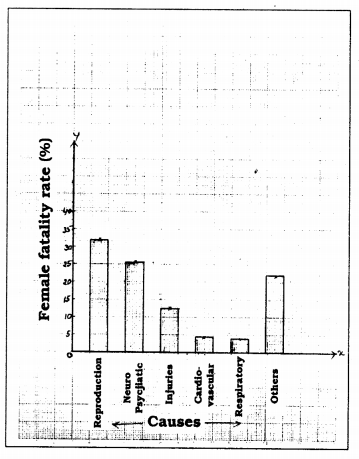(ii) Reproductive health conditions.
(iii) Nerve and skin.

Question 2.
The following data on the number of girls (to the nearest ten) per thousand boys In different sections of Indian society is given below.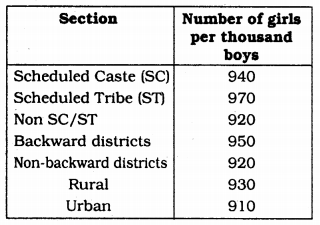(i) Represent the information above by a bar graph.
(ii) In the classroom discuss what conclusions can be arrived at from the graph.
Solution:
(i) Scale: x axis ➝ 1 horizontal = 1 cm.
y axis ➝ 100 girls = 1 cm.(ii) After verification we came to know that Strength of Girls is more in ST section and it is less in Urban section.

Question 3.
Given below are the seats won by different political parties in the polling outcome of a state assembly elections :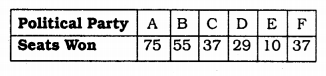(i) Draw a bar graph to represent the polling results.
(ii) Which political party won the maximum number of seats ?
(ii) A Political Party won the maximum number of seats.
Solution:
(i) Scale: x axis ➝ 1 horizontal(Party) = 1 cm.
y axis ➝ 10 Parties = 1 cm.(ii) ‘A’ political Party won the maximum number of seats.

Question 4.
The length of 40 leaves of a plant are measured correct to one millimetre, and the obtained data is represented in the following table:(i) Draw a histogram to represent the given data. [Hint: First make the class intervals continuous]
(ii) Is there any other suitable graphical representation for the same data ?
(iii) Is it correct to conclude that the maximum number of leaves are 153 mm long ? Why ?
Solution:
(i) Scale: x axis ➝ 1 Class interval = 1 cm.
y axis ➝ 2 leaves = 1 cm.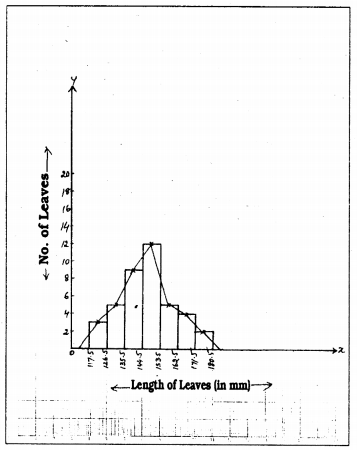(ii) Frequency Polygon.
(iii) No. Because from 145 to 153, length of leaves is 153.

Question 5.
The following table gives the life times of 400 neon lamps: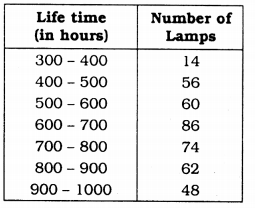(i) Represent the given information with the help of a histogram.
(ii) How many lamps have a life time of more than 700 hours ?
Solution:
(i) Scale: x axis ➝ 1 Class interval = 1 cm.
y axis ➝ 10 Bulbs = 1 cm.(ii) 184 lamps have a life time of more than 700 hours.

Question 6.
The following table gives the distribution of students of two sections according to the marks obtained by them :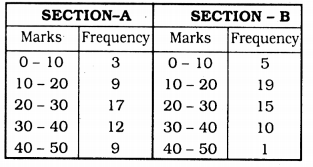Represent the marks of the students of both the sections on the same graph by two frequency polygons. From the two polygons compare the performance of the two sections.
Solution:
(i) Scale: x axis ➝ 5 marks = 1 cm.
y axis ➝ 2 frequency = 1 cm.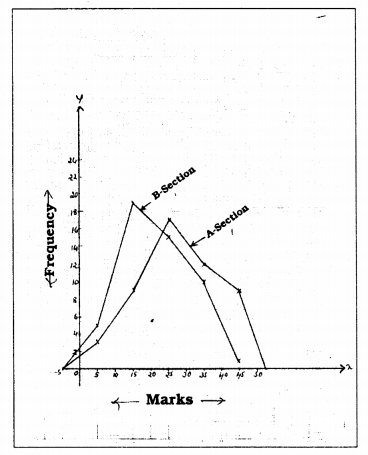Section B students scored less marks in great numbers.

Question 7.
The runs scored by two teams A and B on the first 60 balls in a cricket match are given below :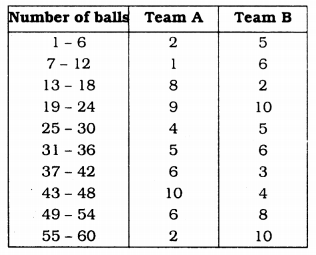Represent the data of both the teams on the same graph by frequency polygons.
(Hint: First make the class intervals continuous.)
Solution:
Class interval is continuous,
1 – 6, 7 – 12 Here the difference is 1.
∴ $$\frac{1}{2}$$ = 0.5 is lower limit is subtracted,
0.5 is taken upper limit, it becomes
0.5 – 6.5
6.5 – 12.5.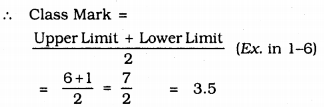Scale: x-axis ➝ 3.5 mark = 1 cm.
y – axis ➝ 1 frequency = 1 cmQuestion 8.
A random survey of the number of children of various age groups playing in a park was found as follows :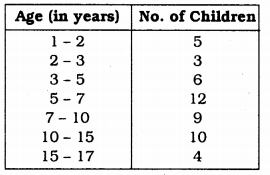Draw a histogram to represent the data above.
Solution:
Scale: x-axis ➝ 1 year = 1 cm.
y – axis ➝ 1 child = 1 cm.Question 9.
100 surnames were randomly picked up from a local telephone directory and a frequency distribution of the number of letters in the English alphabet in the surnames was found as follows :(i) Draw a histogram to depict the given information.
(ii) Write the class interval in which the maximum number of surnames lie
Solution:
(i) Scale: x-axis ➝ 1 letter = 1 cm.
y – axis ➝ 4 surnames = 1 cm.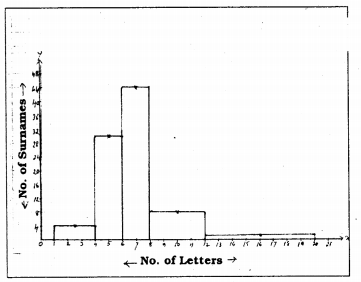(ii) Class interval which has maximum number of surnames is : 6 – 8.

We hope the KSEEB Solutions for Class 9 Maths Chapter 14 Statistics Ex 14.3 help you. If you have any query regarding Karnataka Board Class 9 Maths Chapter 14 Statistics Exercise 14.3, drop a comment below and we will get back to you at the earliest.

error: Content is protected !!